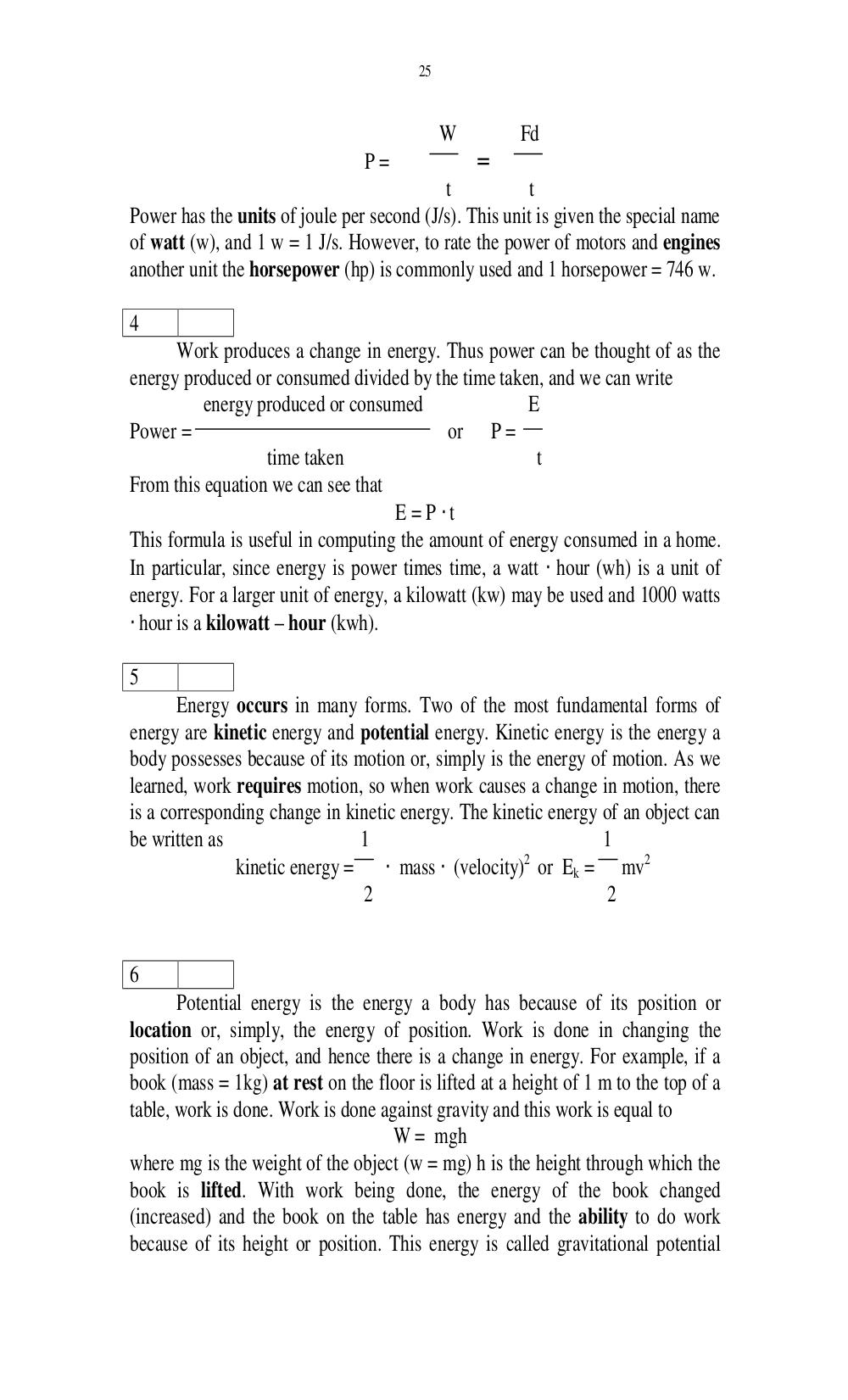# Английский язык. Ильичева Н.А - 25 стр.

Составители:

Рубрика:

• ## Иностранный язык25
W Fd
P = =
t t
Power has the units of joule per second (J/s). This unit is given the special name
of watt (w), and 1 w = 1 J/s. However, to rate the power of motors and engines
another unit the horsepower (hp) is commonly used and 1 horsepower = 746 w.
4
Work produces a change in energy. Thus power can be thought of as the
energy produced or consumed divided by the time taken, and we can write
energy produced or consumed E
Power = or P =
time taken t
From this equation we can see that
E = P t
This formula is useful in computing the amount of energy consumed in a home.
In particular, since energy is power times time, a watt hour (wh) is a unit of
energy. For a larger unit of energy, a kilowatt (kw) may be used and 1000 watts
hour is a kilowatt hour (kwh).
5
Energy occurs in many forms. Two of the most fundamental forms of
energy are kinetic energy and potential energy. Kinetic energy is the energy a
body possesses because of its motion or, simply is the energy of motion. As we
learned, work requires motion, so when work causes a change in motion, there
is a corresponding change in kinetic energy. The kinetic energy of an object can
be written as 1 1
kinetic energy = mass (velocity)
2
or E
k
= mv
2
2 2
6
Potential energy is the energy a body has because of its position or
location or, simply, the energy of position. Work is done in changing the
position of an object, and hence there is a change in energy. For example, if a
book (mass = 1kg) at rest on the floor is lifted at a height of 1 m to the top of a
table, work is done. Work is done against gravity and this work is equal to
W = mgh
where mg is the weight of the object (w = mg) h is the height through which the
book is lifted. With work being done, the energy of the book changed
(increased) and the book on the table has energy and the ability to do work
because of its height or position. This energy is called gravitational potential25

W        Fd
P=               =
t           t
Power has the units of joule per second (J/s). This unit is given the special name
of watt (w), and 1 w = 1 J/s. However, to rate the power of motors and engines
another unit the horsepower (hp) is commonly used and 1 horsepower = 746 w.

4
Work produces a change in energy. Thus power can be thought of as the
energy produced or consumed divided by the time taken, and we can write
energy produced or consumed                E
Power =                                     or    P=
time taken                         t
From this equation we can see that
E = P ⋅t
This formula is useful in computing the amount of energy consumed in a home.
In particular, since energy is power times time, a watt ⋅ hour (wh) is a unit of
energy. For a larger unit of energy, a kilowatt (kw) may be used and 1000 watts
⋅ hour is a kilowatt – hour (kwh).

5
Energy occurs in many forms. Two of the most fundamental forms of
energy are kinetic energy and potential energy. Kinetic energy is the energy a
body possesses because of its motion or, simply is the energy of motion. As we
learned, work requires motion, so when work causes a change in motion, there
is a corresponding change in kinetic energy. The kinetic energy of an object can
be written as                  1                                1
kinetic energy =     ⋅ mass ⋅ (velocity) or Ek = mv2
2

2                                2

6
Potential energy is the energy a body has because of its position or
location or, simply, the energy of position. Work is done in changing the
position of an object, and hence there is a change in energy. For example, if a
book (mass = 1kg) at rest on the floor is lifted at a height of 1 m to the top of a
table, work is done. Work is done against gravity and this work is equal to
W = mgh
where mg is the weight of the object (w = mg) h is the height through which the
book is lifted. With work being done, the energy of the book changed
(increased) and the book on the table has energy and the ability to do work
because of its height or position. This energy is called gravitational potential